Main Content

# Add Node Properties to Graph Plot Data Tips

This example shows how to customize `GraphPlot` data tips to display extra node properties of a graph.

### Plot `GraphPlot` object with Data Tip

Create a `GraphPlot` graphics object for a random directed graph. Add an extra node property `wifi` to the graph.

```rng default G = digraph(sprandn(20, 20, 0.05)); G.Nodes.wifi = randi([0 1], 20, 1) == 1; h = plot(G);```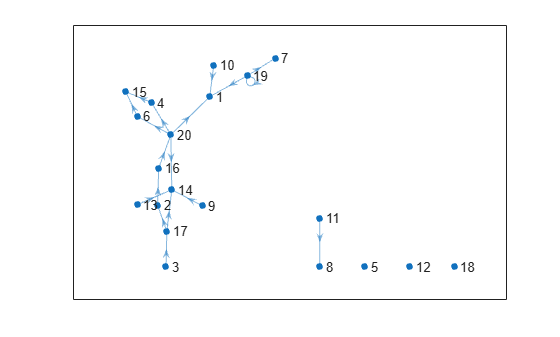Add a data tip to the graph. The data tip enables you to select nodes in the graph plot and view properties of the nodes.

`dt = datatip(h,4,3);`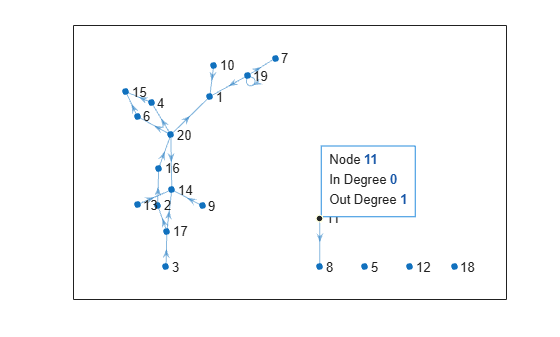By default, the data tips for an undirected graph display the node number and degree. For directed graphs, the display includes the node number, in-degree, and out-degree.

### Customize Existing Data in Data Tip

You can customize the display of data tips for graphics objects by adding, editing, or removing rows of data from the appropriate object properties. For this `GraphPlot` object:

• The `GraphPlot` object handle is `h`.

• The `h.DataTipTemplate` property contains an object that controls the display of the data tips.

• The `h.DataTipTemplate.DataTipRows` property holds the data for the data tips as `DataTipTextRow` objects.

• Each `DataTipTextRow` object has `Label` and `Value` properties. You can adjust the label or data that is displayed in the data tip by modifying these properties.

Change the label for the Node row in the data tip so that it displays as "City".

`h.DataTipTemplate.DataTipRows(1).Label = "City";`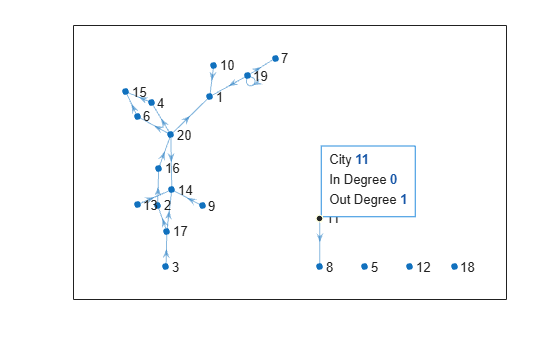The data tip now displays a city number.

### Add Data to Data Tip

The `dataTipTextRow` function creates a new row of data as an object that can be inserted into the `DataTipRows` property. Use `dataTipTextRow` to create a new row of data for the data tip labeled "WiFi" that references the values in the `G.Nodes.wifi` property of the graph. Add this data tip row to the `DataTipRows` property as the last row.

```row = dataTipTextRow('WiFi',G.Nodes.wifi); h.DataTipTemplate.DataTipRows(end+1) = row;```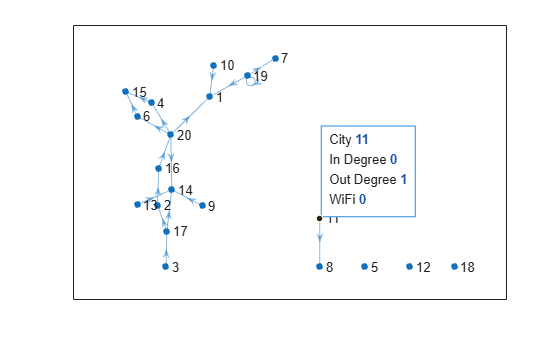The data tip display now includes a WiFi value for each node.

### Remove Data from Data Tip

To remove rows of data from the data tip, you can index into the `DataTipRows` property and assign the rows an empty matrix `[]`. This is the same method you might use to delete rows or columns from a matrix.

Delete the in-degree and out-degree rows from the data tip. Since these appear as the second and third rows in the data tip display, they correspond to the second and third rows of the `DataTipRows` property.

`h.DataTipTemplate.DataTipRows(2:3) = [];`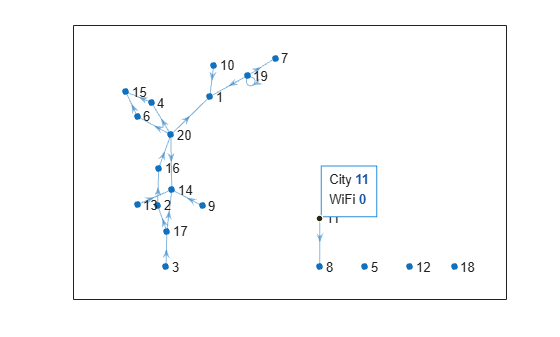The data tip display now only displays the city number and WiFi status.

Download ebook